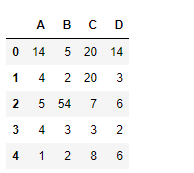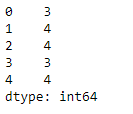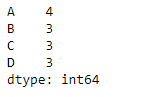Open in App
Not now

# Python | Pandas dataframe.nunique()

• Last Updated : 22 Nov, 2018

Python is a great language for doing data analysis, primarily because of the fantastic ecosystem of data-centric python packages. Pandas is one of those packages and makes importing and analyzing data much easier.

Pandas` dataframe.nunique()` function return Series with number of distinct observations over requested axis. If we set the value of axis to be 0, then it finds the total number of unique observations over the index axis. If we set the value of axis to be 1, then it find the total number of unique observations over the column axis. It also provides the feature to exclude the `NaN` values from the count of unique numbers.

Syntax: DataFrame.nunique(axis=0, dropna=True)

Parameters :
axis : {0 or ‘index’, 1 or ‘columns’}, default 0
dropna : Don’t include NaN in the counts.

Returns : nunique : Series

Example #1: Use `nunique()` function to find the number of unique values over the column axis.

 `# importing pandas as pd ` `import` `pandas as pd ` ` `  `# Creating the first dataframe  ` `df ``=` `pd.DataFrame({``"A"``:[``14``, ``4``, ``5``, ``4``, ``1``], ` `                   ``"B"``:[``5``, ``2``, ``54``, ``3``, ``2``], ` `                   ``"C"``:[``20``, ``20``, ``7``, ``3``, ``8``], ` `                    ``"D"``:[``14``, ``3``, ``6``, ``2``, ``6``]}) ` ` `  `# Print the dataframe ` `df `Let’s use the `dataframe.nunique()` function to find the unique values across the column axis.

 `# find unique values ` `df.nunique(axis ``=` `1``) `

Output :As we can see in the output, the function prints the total no. of unique values in each row.

Example #2: Use `nunique()` function to find the number of unique values over the index axis in a dataframe. The dataframe contains `NaN` values.

 `# importing pandas as pd ` `import` `pandas as pd ` ` `  `# Creating the first dataframe  ` `df ``=` `pd.DataFrame({``"A"``:[``"Sandy"``, ``"alex"``, ``"brook"``, ``"kelly"``, np.nan], ` `                   ``"B"``:[np.nan, ``"olivia"``, ``"olivia"``, "``", "``amanda"],  ` `                   ``"C"``:[``20` `+` `5j``, ``20` `+` `5j``, ``7``, ``None``, ``8``], ` `                   ``"D"``:[``14.8``, ``3``, ``None``, ``6``, ``6``]}) ` ` `  `# apply the nunique() function ` `df.nunique(axis ``=` `0``, dropna ``=` `True``) `

Output :The function is treating the empty string as a unique value in column 2.

My Personal Notes arrow_drop_up
Related Articles